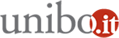# Statistics seminar 2019 - "A challenge for statisticians: the design-based spatial interpolation"

31/01/2019 dalle 11:30 alle 13:30

Dove Dipartimento di Scienze Statistiche - via delle Belle Arti 41 - Aula III - 2° pianoAggiungi l'evento al calendario

Relatore
Lorenzo Fattorini - Università degli Studi di Siena

Abstract
Accurate and updated wall-to wall maps depicting the spatial pattern of ecological and economic attributes throughout the study area represents a crucial information for evaluations, decision making and planning. Traditionally maps, as well as most of the issues of spatial statistics, are approached in a model-based framework (e.g. Cressie 1993). Recently we have attempted to construct maps in a complete design-based framework simply exploiting the inverse distance weighting interpolator and deriving the properties from the characteristics of the sampling scheme adopted. We first approached the problem of making maps for finite population of spatial units, when the survey variable is the amount of an attribute within units (Fattorini et al. 2018a). Subsequently, we considered the problem of making maps for continuous populations when the survey variable is, at least in principle, defined at each point of the continuum representing the study area (Fattorini et al. 2018b). Finally, we have faced the problem of constructing maps for finite populations of marked points.

Our design-based approach to spatial mapping avoids the massive modelling involved in model-based approaches, i.e. the use of spatial models on lattices required for finite populations of spatial units (e.g. Cressie, Chapter 6), the use of second-order stationary spatial processes required for continuous populations (e.g. Cressie, Chapter 3) and the marked point processes in the plane required for finite populations of marked points (e.g. Cressie, Chapter 8). Design-based asymptotic unbiasedness and consistency of the resulting maps are achieved exploiting different asymptotic scenarios for the three cases, at the cost of supposing i) some forms of smoothness of the survey variables throughout the study area; ii) some sort of regularities that are necessary in the case of finite populations such as regularities in the shape of spatial units or regularities in the enlargements of the point populations; iii) asymptotically balanced spatial sampling schemes; iv) the use of distance functions sharing some mathematical properties. It is worth noting that iii) is satisfied by the more common sampling schemes adopted in spatial surveys and iv) does not constitute an assumption because it can be readily ensured by the user.

Organizzatore
Daniela Cocchi